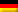The distillate cooler is calculated in such a way that if the return flow into the column is blocked, the complete (maximum) mass flow of overhead product can be cooled.

Cooling water inlet: ϑW1 = 20 °C
Cooling water outlet: ϑW2 = 30 °C

Reference temperature for the specific heat capacity of the cooling water:

ϑWB = (20 °C + 30 °C)/2 = 25 °C

Specific heat capacity (linear interpolated according to table inside rear cover):

cW = 4179 J/(kg · K)

Required heat flow (p. 238):

Φ = qmD · cD · (ϑbD – ϑD2) = 0,258 kg/s · 1740 J/(kg · K) · (80,3 – 40) °C = 18091 W

K value according to table (p. 235): K = 600 W/(m² – K)

Mean logarithmic temperature difference (p. 237):

Required exchange area (p. 239):

Dieser Beitrag ist auch verfügbar auf:Deutsch (German)